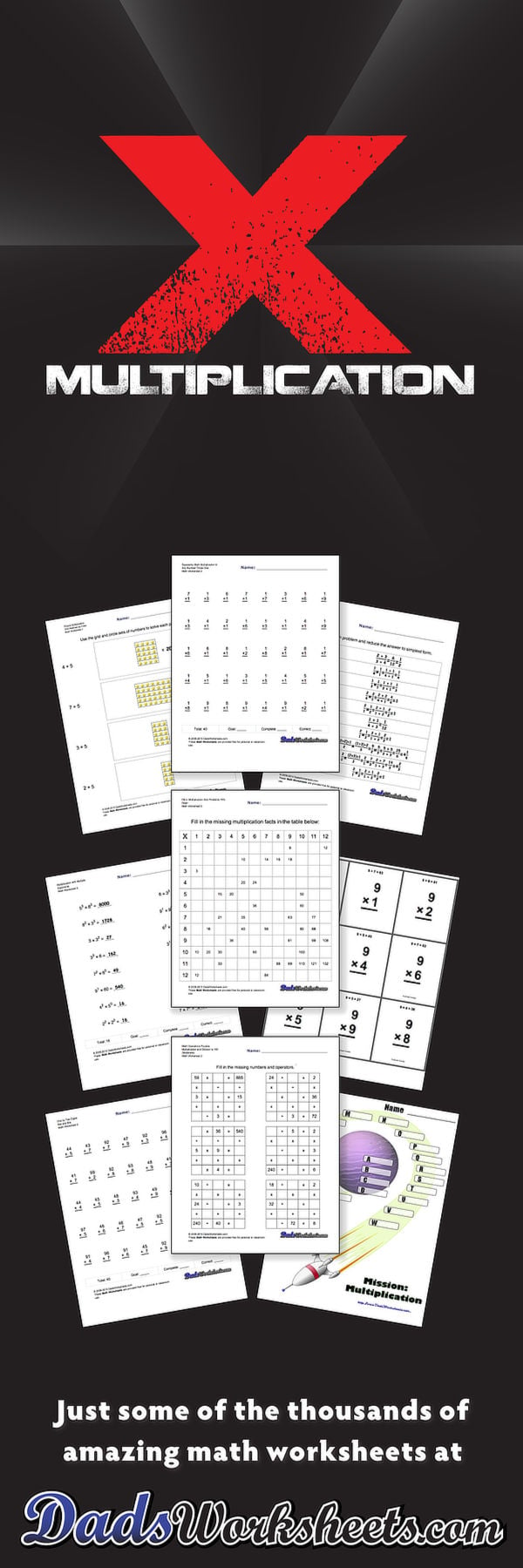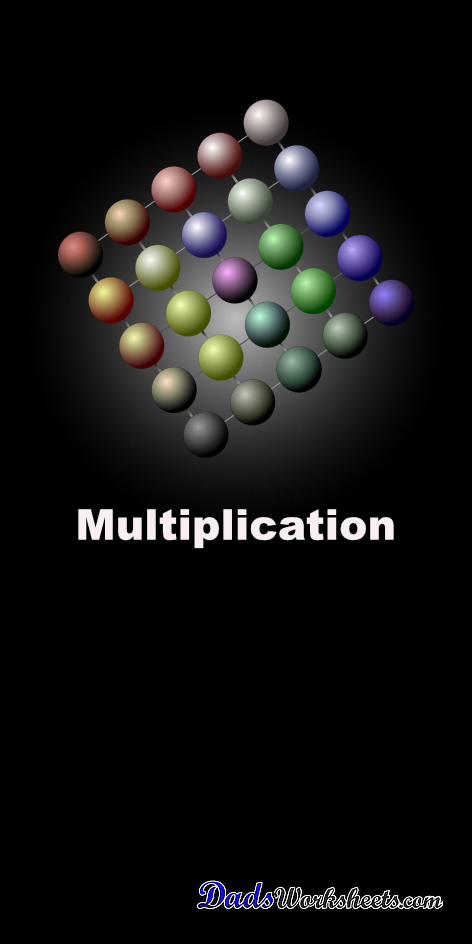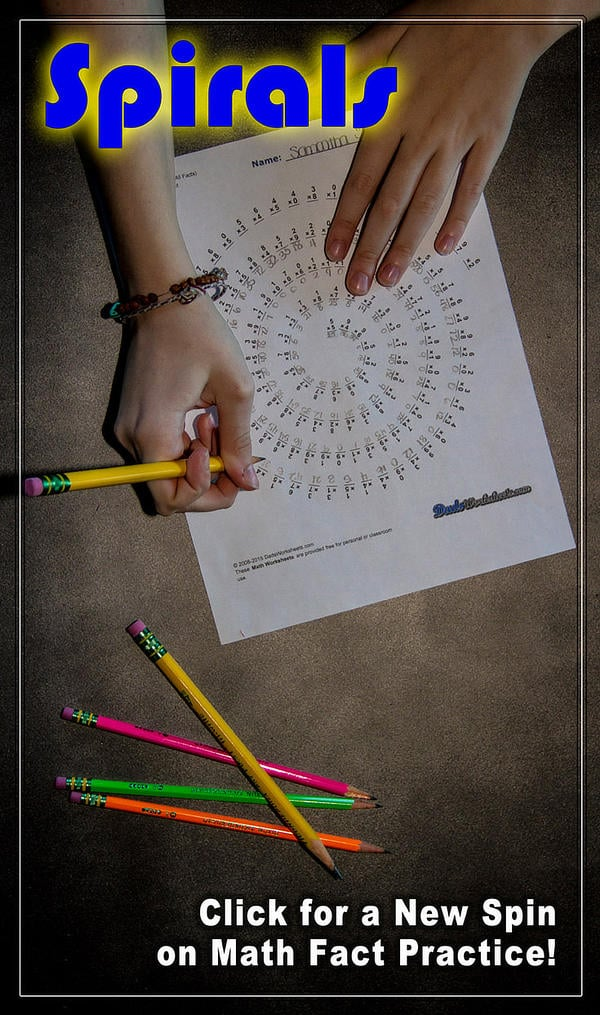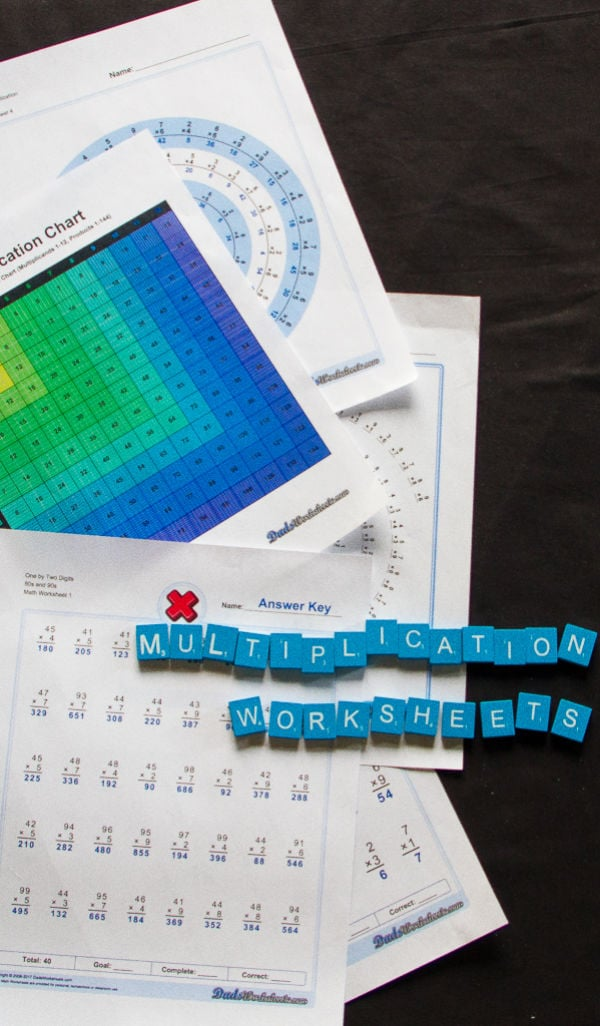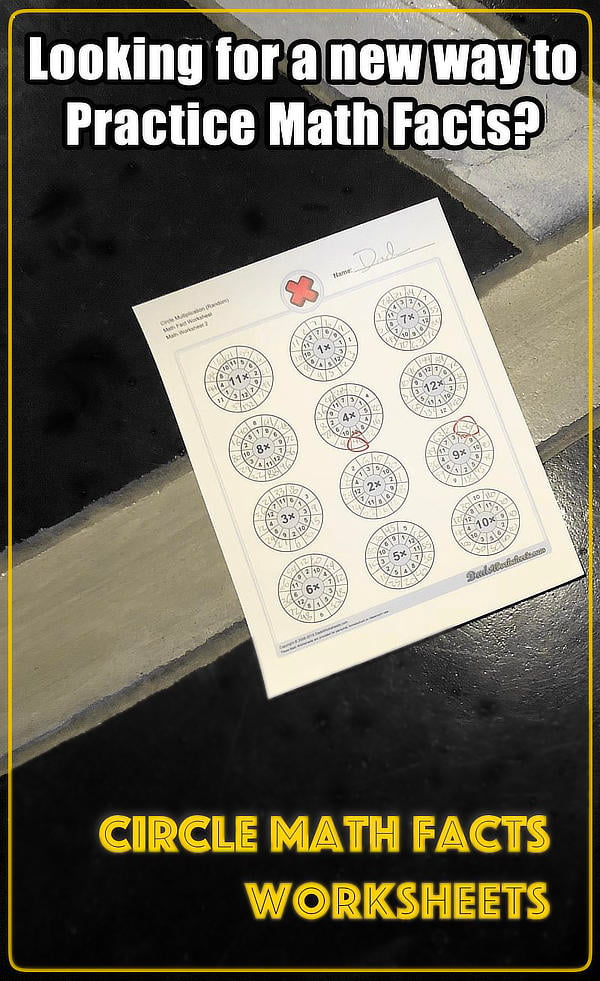One Dad. Four daughters. 9,251 worksheets... and counting!

# Multiplication Worksheets

These multiplication worksheets include timed math fact drills, fill-in multiplication tables, multiple-digit multiplication, multiplication with decimals and much more! And Dad has a strategy for learning those multiplication facts that you don't want to miss. When you're done, be sure to check out the unique spiral and bullseye multiplication worksheets to get a completely different spin on your math fact practice!

## One Minute Timed Multiplication Worksheets

### 96 Multiplication Worksheets

These basic math fact multiplication worksheets are similar to the RocketMath, Mad Math Minutes or Mastering Math Facts multiplication programs used at many schools. These are typically one minute, timed tests (Try this countdown timer!)

## Two Minute Timed Multiplication Worksheets

### 48 Multiplication Worksheets

80 and 100 problem Spaceship Math/RocketMath/Mad Minute timed multiplication worksheets. These are great for multiplication practice at home if your school is using a one minute timed multiplication worksheet... They make a one minute timed test seem easy!

## Rules for Mastering the Times Tables Worksheets

### 32 Multiplication Worksheets

These practice multiplication worksheets support the concepts in Dad's Eight Simple Rules for Learning Multiplication and provide an alternative to rote memorization of the times table. This is a great series of multiplication worksheets to incrementally master the times tables using just a handful of rules.

## Circle Multiplication Facts Worksheets

### 40 Multiplication Worksheets

These multiplication worksheets emphasize groups of related facts and there are variations with the facts in order to facilitate skip counting, or with random products that help facilitate fact memorization. Try the variations with all facts, or print the worksheets that focus only on specific families of multiplication facts that need more practice!

## Spiral Multiplication Facts Worksheets

### 44 Multiplication Worksheets

Tired of the same old math fact worksheets with rows and rows of problems? These multiplication worksheets present the facts in a spiral layout that provides fun a twist on memorizing the times tables. They use the same fact layouts as the spaceship math sheets above, so try the first two sets of worksheets if you are looking for all of the multiplication facts or for practice without the easier problems, or try the others in series for an incremental approach to learning the facts.

## Bullseye Multiplication

### 8 Multiplication Worksheets

Get on target with those multiplication math facts! These fun multiplication worksheets provide some additional kinesthetics over and above your usual math fact practice. If you are looking for a fun or interesting practice worksheet, or just need to change things up a bit, these unique multiplication worksheets are exactly what you need!

## Multiplication with x10, x11 and x12 Facts

### 40 Multiplication Worksheets

These multiplication worksheets extend the Spaceship Math one minute timed tests with the x10, x11 and x12 facts. Even if your school isn't practicing multiplication past single digits, these are valuable multiplication facts to learn for many time and geometry problems.

## Multiplication Grid

### 36 Multiplication Worksheets

Grid problem multiplication worksheets for introducing multiplication and discovering patterns in multiplication problems (Answer keys to these sheets make a great multiplication fact chart!)

## Visual Multiplication Worksheets

### 20 Multiplication Worksheets

A good way to start teaching multiplication facts is to provide some sort of visual or manipulative aide to assist with counting strategies for solving basic multiplication facts. These visual multiplication worksheets have arrays of blocks that allow students to count rows, columns and totals to build foundational concepts of how multiplication works.

## Conventional Multiplication Practice Worksheets

### 64 Multiplication Worksheets

Conventional times table math worksheets. These multiplication worksheets introduce math facts in order, as opposed to by fact families. If you grew up more than 20 years ago doing timed multiplication tests, these may look familiar....

## Multiplication with Times 12 Practice Worksheets

### 48 Multiplication Worksheets

Conventional times table practice with values through x12, similar to the timed multiplication worksheets above. One minute timed test layout.

## Conventional Two Minute Multiplication Tests

### 72 Multiplication Worksheets

Conventional times tables worksheets setup for 100 problem, two minute timed practice. Timed tests are great for keeping your multiplication facts in shape, especially over the summer break. These are similar to the multiplication worksheets I had to do as a kid in Oregon. But I am going to say, even better.

## Progressive Times Table Worksheets

### 48 Multiplication Worksheets

These are progressive multiplication worksheets for incrementally building multiplication facts, with facts grouped by a common multiplicand. Start with the ones time table, and then progress through the two times table, three times table, etcetera, all the way through the nine times table. 40 problem timed test format.

## Progressive Times Table Worksheets with Times 12

### 48 Multiplication Worksheets

Progressive practice multiplication worksheets including the twelve times table. These worksheets are perfect for one minute timed tests.

## Special Series Multiplication Worksheets

### 12 Multiplication Worksheets

Squares and binary progression multiplication worksheets. These are common multiplication facts worth memorizing.

## Multiple Digit Multiplication Worksheets

### 68 Multiplication Worksheets

These multiplication worksheets introduce two digit and three digit multiplication problems. Try out this multiplication calculator to see interactively how to do long multiplication.

## Zeroes in Tens Place Worksheets

### 16 Multiplication Worksheets

These multiplication worksheets introduce multiple digit problems that highlight a particularly confusing scenario when multiplying place values with a zero in them. Give them a try if you are having trouble keeping track of the adjusted place values in the partial products and they will give your multiplication skills a real workout.

## Multiplication with Decimals Worksheets

### 32 Multiplication Worksheets

Multiplication worksheets for multiplication with decimals. Like multiple digit multiplication, but with an irritating little dot bouncing around to keep you on your toes.

## Factors of Ten Multiplication Worksheets

### 24 Multiplication Worksheets

Multiplication worksheets for learning multiplication by factors of 10. This is a simple multiplication trick, but still one worth practicing if you just picked it up.

## Money Multiplication Worksheets

### 48 Multiplication Worksheets

Multiplication worksheets that multiply dollars times whole and decimal unit quantities. Some results will require rounding.

## A Guide to the Multiplication Worksheets

Welcome to the multiplication worksheets page, repleat with that pesky x shaped symbol shared with us by the ancient Babylonians, who thankfully kept their crazy base 60 numbering system to themselves. Well except for when we get into measuring angles in degrees and units of time. Let's ignore that for now. Lousy Babylonians.

Multiplication is one of the four elementary operations, and one often challenging to learn because most of the facts cannot simply be 'counted up' or 'counted down' the way that addition or subtraction facts are solved. Because of this, many strategies for learning multiplication have evolved. This page contains multiplication worksheets that address many of these techniques for mastering the times tables, some of which may correspond to the approaches being used in your classroom or homeschool curriculum. Most students will start learning multiplication concepts in third grade, and by the end of 4th grade the times table facts through x10 should be memorized.

I provide my own rules for learning multiplication and one of the multiplication worksheet sets below focuses on that approach. But often, brute force memorization of the multiplication facts is the most obvious and fastest approach, and for better or worse, is usally the way multiplication proficiency is measured. There are various versions of timed multiplication drills, including one minute and two minute timed worksheets. You will find incremental or progressive approaches, where facts are gradually introduced and repeated. Or, look for the conventional series of worksheets which focus strictly on one set of multiplication facts at a time (for example, all the x7 facts) to the exclusion of any of the others. That's how I learned them way too many years ago in a tiny school in Beavercreek, Oregon.

A tactic taken by many schools is to have students take complete a timed multiplication worksheet once per day. The multiplication worksheets used for this approach start with a few basic facts, and as the student is able to pass these tests with high levels of accuracy in one or two minutes, they advance to the next level and new multiplication facts are introduced. There are different names for these approaches including Mad Minute tests or Rocket Math, but the basic incremental strategy coupled with a daily multiplication timed test is very common.You will find different sets of timed multiplication worksheets here that should be suitable for at home practice or more structured classroom use.

No matter what tactic you take to mastering the multiplication facts, the results will come to bear when dealing with more advanced topics including multiple digit multiplication, multiplying decimals, squares, cubes and money problems.You will find multiplication worksheets on all of these topics and more in this section.

The multiplication worksheets below also include several sets of multi-digit multiplication worksheets, including ones that focus on some of the more difficult steps for multiplying two and three digit numbers.

Good luck and get multipying!

Looking for more resources for getting your multiplication skills in order? Try these resources, worksheets and strategies: